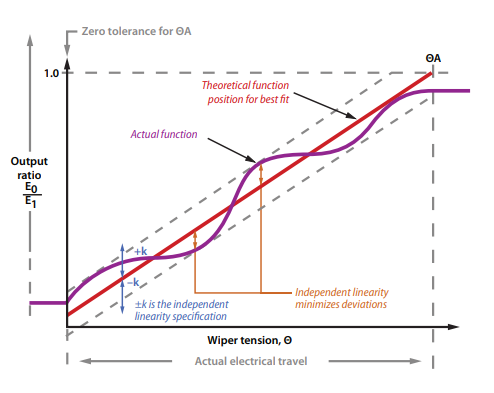# Potentiometer Linearity Tolerance

There are 4 ways to explain potentiometer linearity. Firstly, Linearity is the difference between the output voltage and mechanical rotation from the shaft.

1. Absolute - the actual electrical angle of the potentiometer

2. Independent - most commonly specified linearity for industrial applications that minimize deviations

3. Zero Based - This is the independent linearity where the zero travel end of the theoretical reference line extends over the actual electrical travel. In this graph, the reference line extends over the actual electrical travel.

4. Terminal Based - It’s the maximum deviation of the actual output from a straight reference line drawn through minimum and maximum end points.Content and Graph compliments of Bourns_pot_linearity_technote.pdf and Bourns® Potentiometers